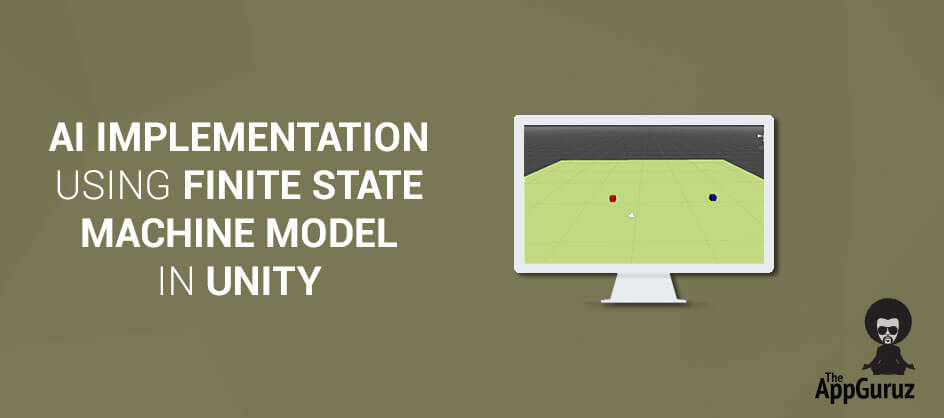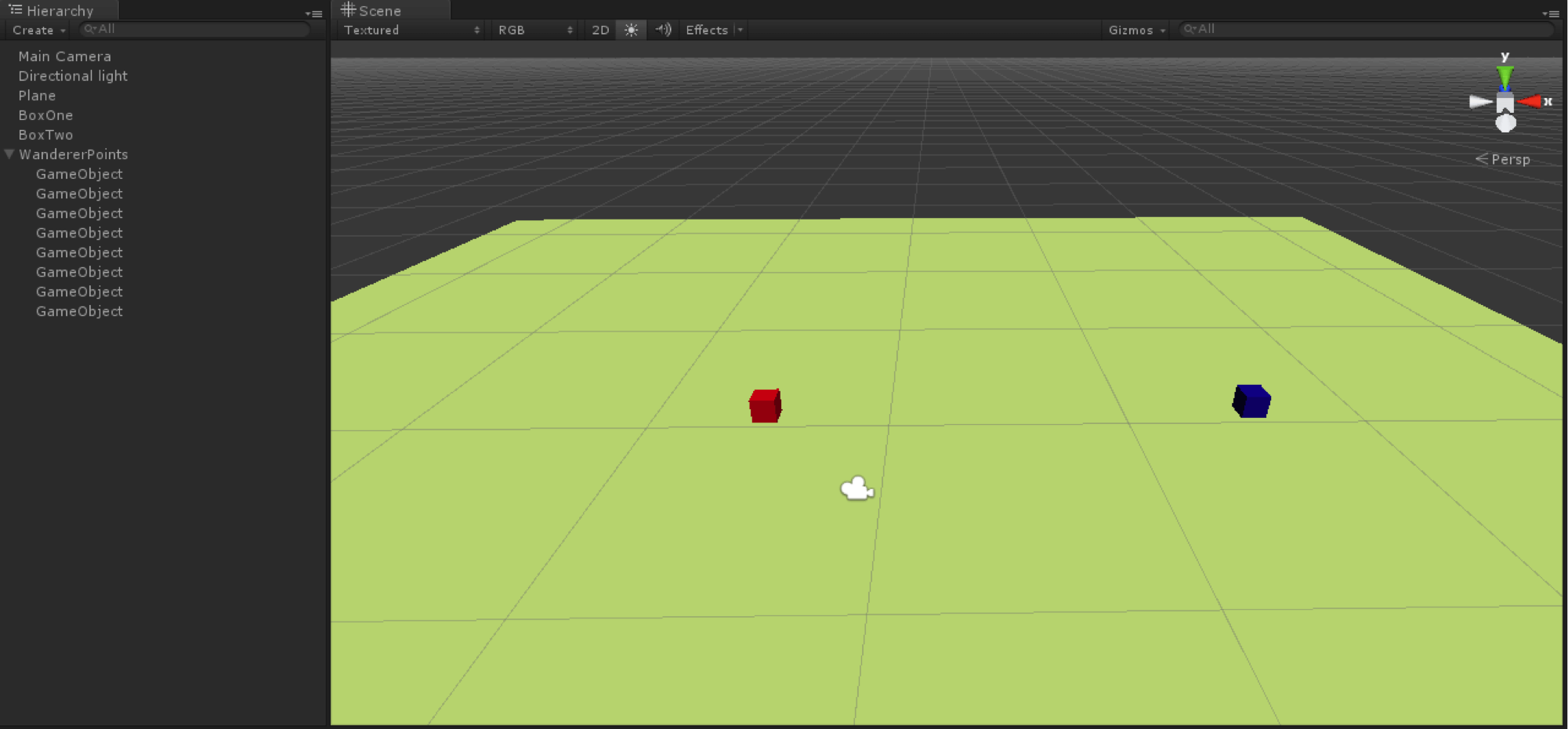## Objective

Main objective of this post is to give you an idea about how to work with FINITE State Machine Model in AI Implementation in Unity.

Basics of FSM:

• Finite State Machine Framework is perfect for implementing AI in games, producing great results without a complex code. It is a model of computation based on a hypothetical machine made of one or more states. Only a single state can be active at a time, so the machine must transition from one state to another in order to perform different actions.

• Finite State Machine Frameworks are commonly used to manage, organize and represent an execution flow of different states, which is very useful in implementing AI in games. The "brain" of an enemy, for instance, can be implemented using a FSM: every state represents an action, such as Patrol, Chase, Evade or Shoot or any other kind of actions.

• AI FSMs work same as Unity's Animation FSMs, where one animation state changes to another as per the requirement. AI FSM can be implemented either by using third party plug-ins like Behave or it can be implemented directly by scripting as well.

To get a basic Idea of Finite State Machine Framework, we will implement one tutorial in the simplest way, using the switch statements. Then we’ll study how to use a framework to make the AI implementations easier to manage and extend.

Follow the below steps to implement simple FSM.

Here we will have two Boxes, where one box will be controlled by the player and the other will be AI controlled. This AI controlled box will be either in chasing state or in patrolling state, i.e. the box will start chasing player box, once the player box comes in the proximity of the AI controlled box. And it will switch back to patrol state if the player evades far enough from AI's defined vision.

## Step 1 Set up the scene

Take One Plane and Two Boxes and Set up the scene as shown in the following figure.## Step 2 Create and place Game Objects

Create empty game objects and place it as Wanderer Points. Place these empty game objects around the plane as per your choice. Here the Blue Cube is the AI cube and the Red One is the Player controlled cube. Place them at a distance far enough, so that AI doesn't start following at the start itself.

## Step 3 Implement the BoxMovement Script

Implement the BoxMovement Script to the player controlled box as given below.

``````public class BoxMovementScript : MonoBehaviour
{
public float speed = 0.1f;
private Vector3 positionVector3;
void Update ()
{
InitializePosition ();
if (Input.GetKey (KeyCode.LeftArrow)) {
GoLeft ();
}
if (Input.GetKey (KeyCode.RightArrow)) {
GoRight ();
}
if (Input.GetKey (KeyCode.UpArrow)) {
GoTop ();
}
if (Input.GetKey (KeyCode.DownArrow)) {
GoDown ();
}
RotateNow ();
}
private void InitializePosition ()
{
positionVector3 = transform.position;
}
private void RotateNow ()
{
Quaternion targetRotation = Quaternion.LookRotation (transform.position - positionVector3);
transform.rotation = targetRotation;
}
private void GoLeft ()
{
transform.position = transform.position + new Vector3 (-speed, 0, 0);
}
private void GoRight ()
{
transform.position = transform.position + new Vector3 (speed, 0, 0);
}
private void GoTop ()
{
transform.position = transform.position + new Vector3 (0, 0, speed);
}
private void GoDown ()
{
transform.position = transform.position + new Vector3 (0, 0, -speed);
}
}``````

## Step 4 FSM Modelled Script

Construct an FSM Modelled Script as given below.

``````public class FSM : MonoBehaviour
{
//Player Transform
protected Transform playerTransform;
//Next destination position of the Box
protected Vector3 destPos;
//List of points for patrolling
protected GameObject[] pointList;
protected virtual void Initialize (){
}
protected virtual void FSMUpdate (){
}
protected virtual void FSMFixedUpdate (){
}
void Start ()
{
Initialize ();
}
void Update ()
{
FSMUpdate ();
}
void FixedUpdate ()
{
FSMFixedUpdate ();
}
}
``````

## Step 5 AI Script for the Box

Construct an AI Script for the Box that extends the Above FSM Modelled script.

``````public class BoxFSM : FSM
{
public enum FSMState
{
None,
Patrol,
Chase,
}
//Current state that the Box is in
public FSMState curState;
//Speed of the Box
private float curSpeed;
//Box Rotation Speed
private float curRotSpeed;

//Initialize the Finite state machine for the  AI Driven Box
protected override void Initialize ()
{
curState = FSMState.Patrol;
curSpeed = 5.0f;
curRotSpeed = 1.5f;

//Get the list of points
pointList = GameObject.FindGameObjectsWithTag ("WandarPoint");

//Set Random destination point for the patrol state first
FindNextPoint ();

//Get the target enemy(Player)
GameObject objPlayer = GameObject.FindGameObjectWithTag ("Player");
playerTransform = objPlayer.transform;

if (!playerTransform)
print ("Player doesn't exist.. Please add one " + "with Tag named 'Player'");

}

//Update each frame
protected override void FSMUpdate ()
{
switch (curState) {
case FSMState.Patrol:
UpdatePatrolState ();
break;
case FSMState.Chase:
UpdateChaseState ();
break;
}
}

protected void UpdatePatrolState ()
{
//Find another random patrol point on reaching the current Point
//point is reached
if (Vector3.Distance (transform.position, destPos) <= 2.5f) {
print ("Reached to the destination point\n" + "calculating the next point");
FindNextPoint ();
}

//Check the distance with player Box
//When the distance is near, transition to chase state
else if (Vector3.Distance (transform.position, playerTransform.position) <= 15.0f) {
print ("Switch to Chase State");
curState = FSMState.Chase;
}

//Rotate to the target point
Quaternion targetRotation = Quaternion.LookRotation (destPos - transform.position);
transform.rotation = Quaternion.Slerp (transform.rotation, targetRotation, Time.deltaTime * curRotSpeed);

//Go Forward
transform.Translate (Vector3.forward * Time.deltaTime * curSpeed);
}

protected void FindNextPoint ()
{
print ("Finding next point");
int rndIndex = Random.Range (0, pointList.Length);
Vector3 rndPosition = Vector3.zero;
destPos = pointList [rndIndex].transform.position + rndPosition;

//Check Range to Move and decide the random point
//as the same as before
if (IsInCurrentRange (destPos)) {
destPos = pointList [rndIndex].transform.position + rndPosition;
}
}

protected bool IsInCurrentRange (Vector3 pos)
{
float xPos = Mathf.Abs (pos.x - transform.position.x);
float zPos = Mathf.Abs (pos.z - transform.position.z);
if (xPos <= 8 && zPos <= 8)
return true;
return false;
}

protected void UpdateChaseState ()
{
//Set the target position as the player position
destPos = playerTransform.position;
//Check the distance with player Box When
float dist = Vector3.Distance (transform.position, playerTransform.position);

//Go back to patrol as player is now too far
if (dist >= 15.0f) {
curState = FSMState.Patrol;
FindNextPoint ();
}
//Rotate to the target point
Quaternion targetRotation = Quaternion.LookRotation (destPos - transform.position);
transform.rotation = Quaternion.Slerp (transform.rotation, targetRotation, Time.deltaTime * curRotSpeed);
//Go Forward
transform.Translate (Vector3.forward * Time.deltaTime * curSpeed);
}
}``````

Apply this script to the cube which is going to follow the player, also do not forget to tag the player as Player and wander points as WandarPoint , now as seen in the script the FSMUpdate() method will be called which is overridden in the child class and hence it will be executed on each Update().

Here the switch case is implemented which will be used to execute actions as per the current state. Thus extension of AI is quite simple by just adding new states. Initialize() method is also overridden and will be called on Start() method execution. UpdatePatrolState() will be executed on each update when current state is to patrol around wanderer points and same will happen for UpdateChaseState() , when the player comes in proximity of the AI Box.

State Change is checked at every Update, if when patrolling, the player comes in the vision of the AI Box, the state will be changed to patrolling and same kind of check is kept in chase mode to check if player has moved out of its vision, then switch back to patrol state.

Conclusion:

FSM's are quite easy to understand and implement, FSMs can be used for implementing complex AI decisions states with quite simplicity. They can also be represented using a graph, which allows a developer to understand easily and hence the developer can tweak, change and optimize the final result.

The implementation of a FSM using functions or methods to represent states is simple, powerful and easily extensible. Even more complex AI can be applied using a stack-based FSM, which ensures a manageable and stable execution flow without affecting the code negatively. So make your enemies smarter using Finite State Machines and make your games a success.

Got an Idea of Game Development? What are you still waiting for? Contact us now and see the Idea live soon. Our company has been named as one of the best Game Development Company in India.Talented Game Developer as well as a player with a mania for video games and the game industry. Always Ready to take up challenging Projects. Proud to be making with the TheAppGuruz Team